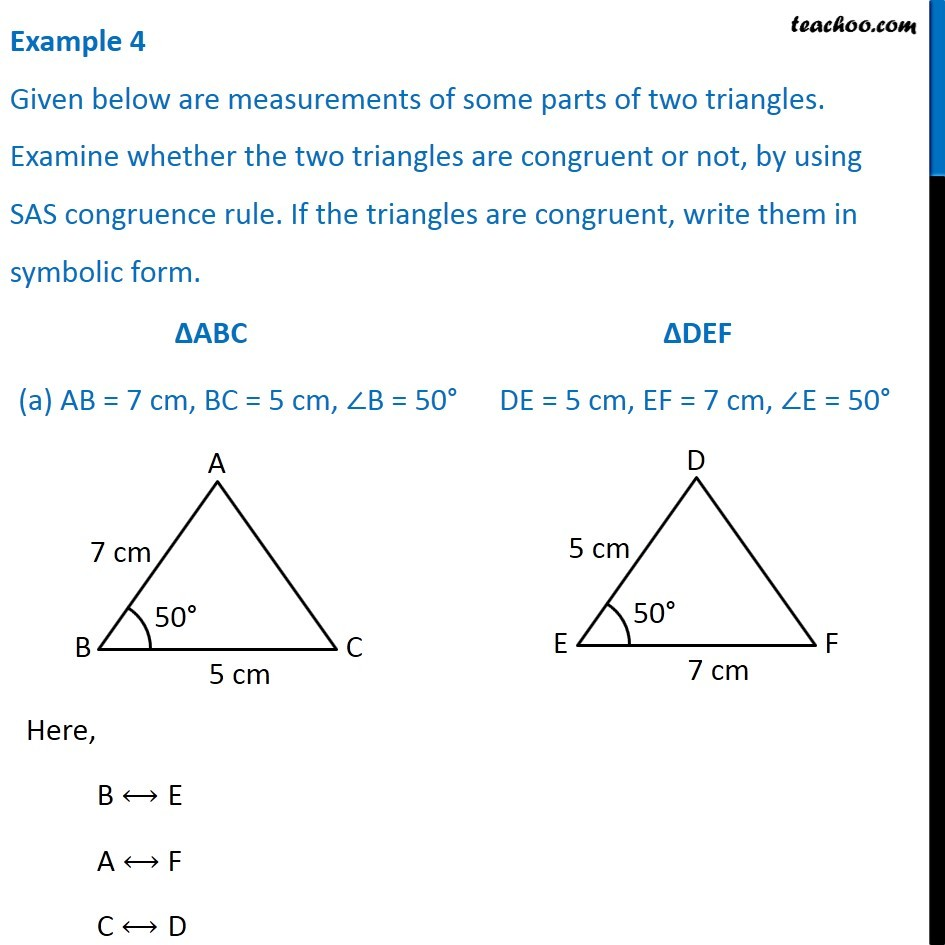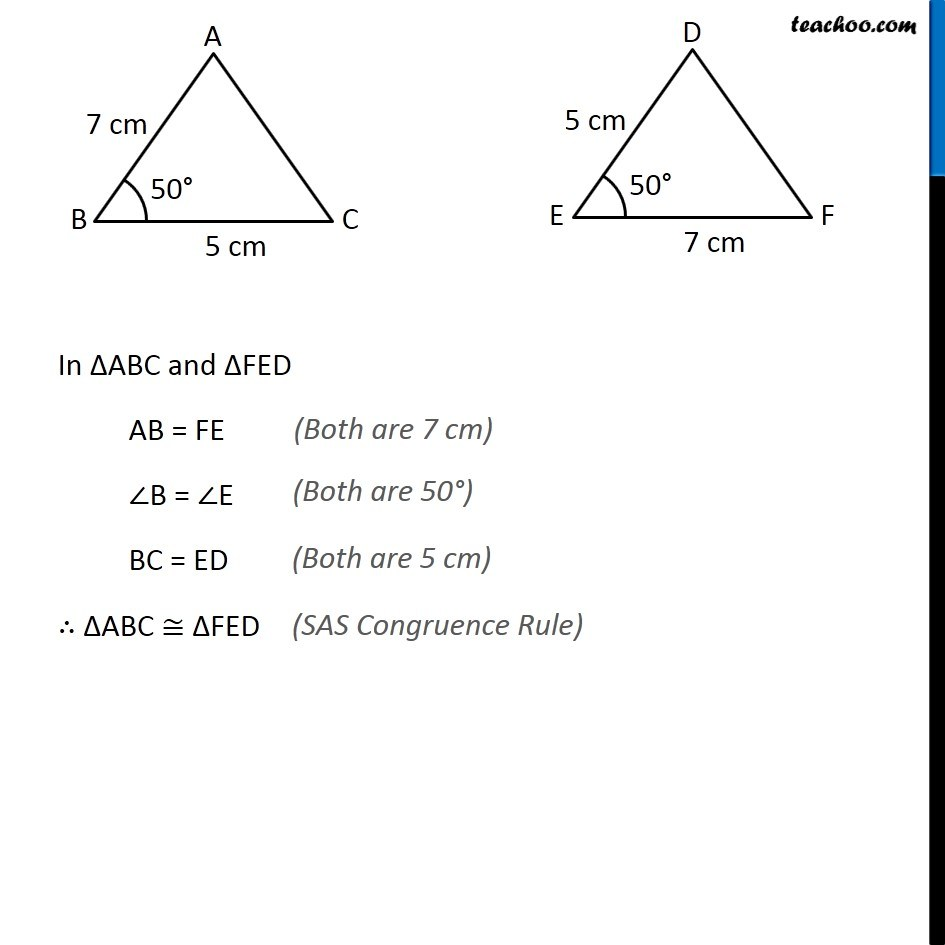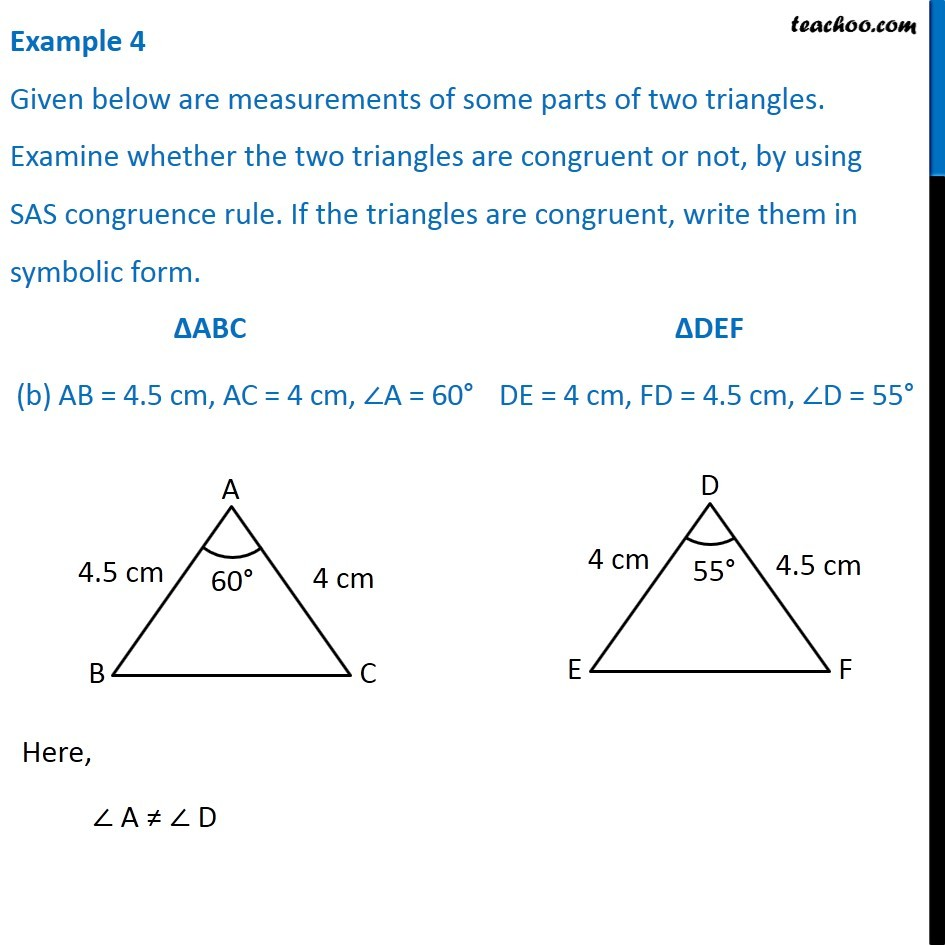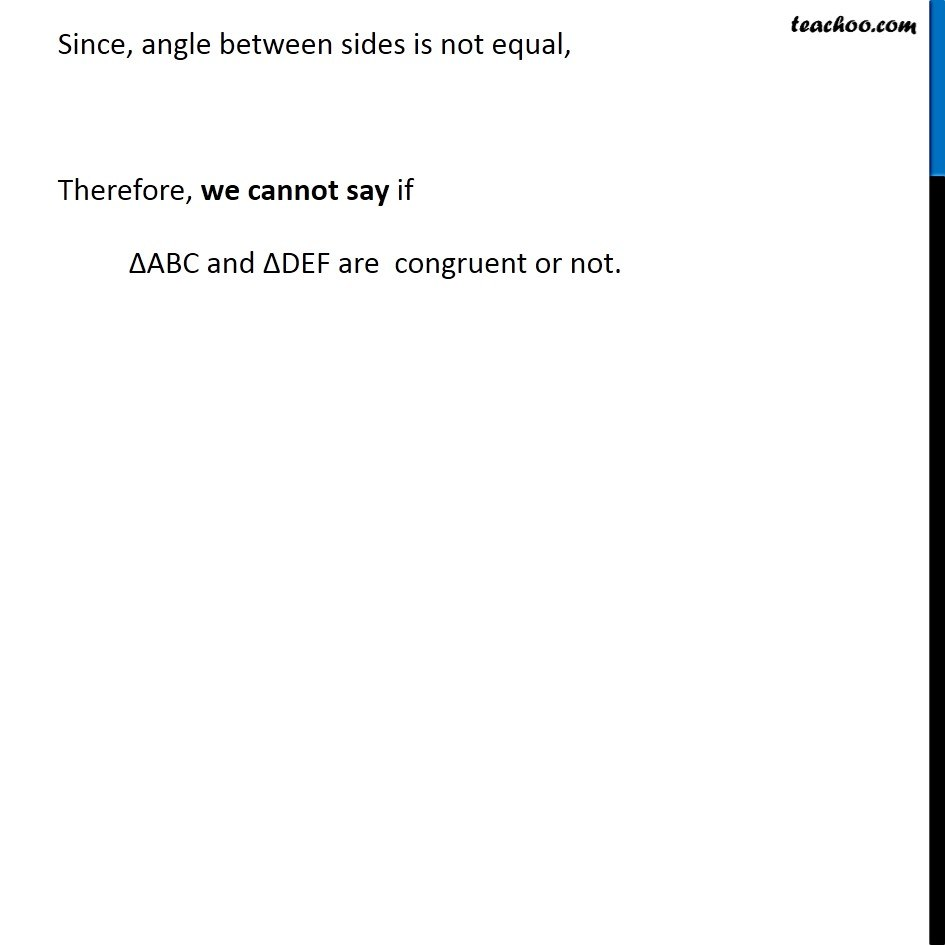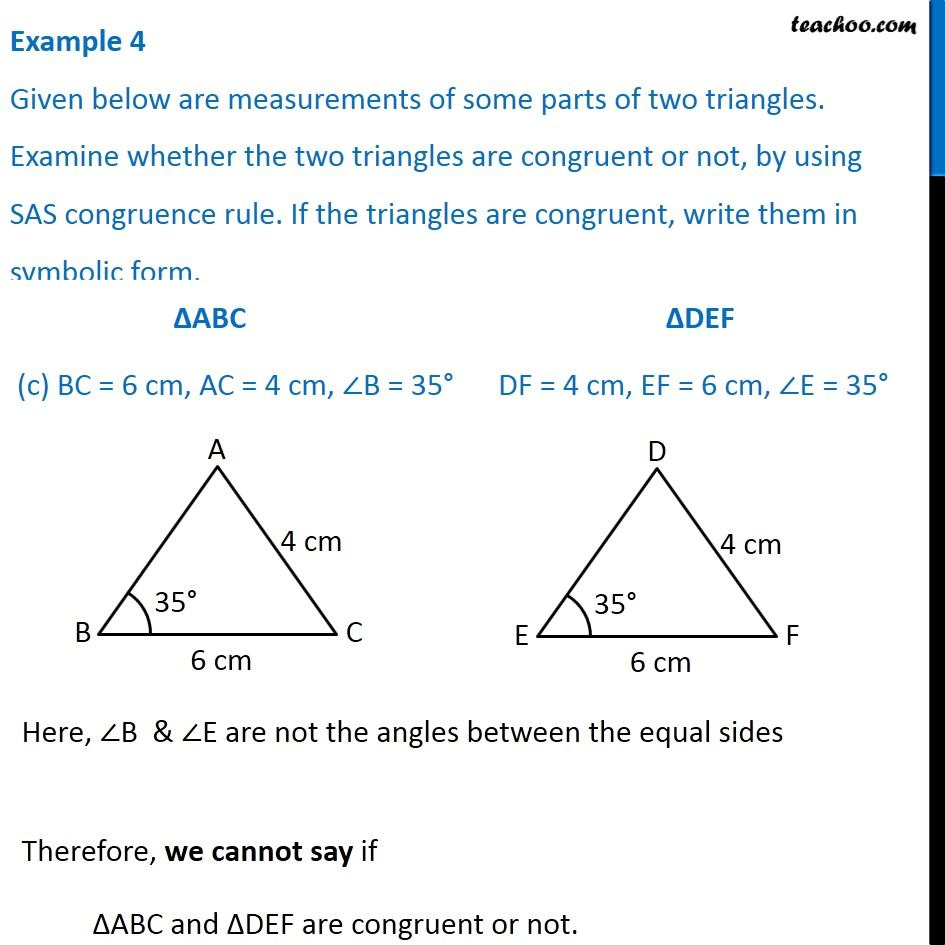Subscribe to our Youtube Channel - https://you.tube/teachoo

1. Chapter 7 Class 7 Congruence of Triangles
2. Serial order wise
3. Examples

Transcript

Example 4 Given below are measurements of some parts of two triangles. Examine whether the two triangles are congruent or not, by using SAS congruence rule. If the triangles are congruent, write them in symbolic form. Here, B ⟷ E A ⟷ F C ⟷ D In ∆ABC and ∆FED AB = FE ∠B = ∠E BC = ED ∴ ∆ABC ≅ ∆FED Example 4 Given below are measurements of some parts of two triangles. Examine whether the two triangles are congruent or not, by using SAS congruence rule. If the triangles are congruent, write them in symbolic form. Here, ∠ A ≠ ∠ D Since, angle between sides is not equal, Therefore, we cannot say if ∆ABC and ∆DEF are congruent or not. Example 4 Given below are measurements of some parts of two triangles. Examine whether the two triangles are congruent or not, by using SAS congruence rule. If the triangles are congruent, write them in symbolic form. Here, ∠B & ∠E are not the angles between the equal sides Therefore, we cannot say if ∆ABC and ∆DEF are congruent or not.

Examples